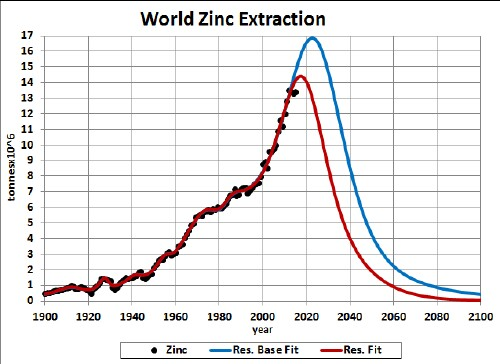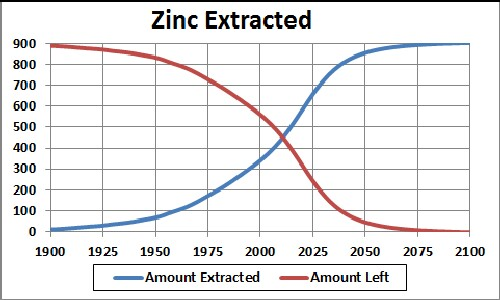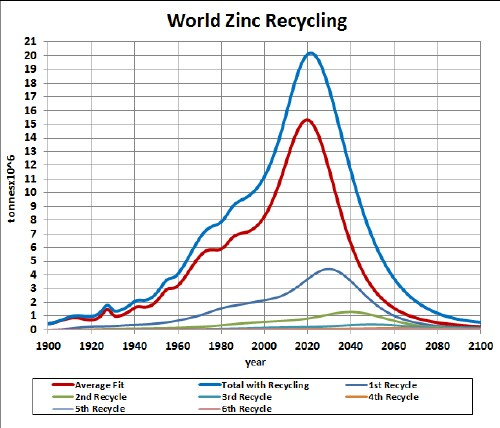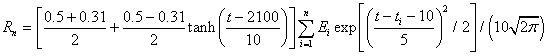# Zinc Depletion

Zinc is used to make high-strength steel alloys and many kinds of batteries. The graph below shows the zinc extraction data for the world and two Verhulst functions fits to the data in order to extrapolate into the future.Zinc extraction rate for the world and Verhulst functions fits to the data.

The red curve is a fit which incorporates the latest reserves estimate (~250 x 106 tonnes), and the blue curve is a fit which incorporates the latest reserves-base estimate (~480 x 106 tonnes).

Taking an average extraction curve of the two fits, the crossover point at ~2011 when the amount extracted is equal to the amount left to be extracted is shown here:## Recycling

Zinc, especially the nickel used in batteries, is recycled to a high degree. Assume that:

• The zinc-extraction curve is the average of the two curves given above.
• Recycling of zinc follows a hyperbolic tangent curve from 31% to 50% recycling with a break point of year 2100 and width 10 years.
• The recycling is delayed by a Gaussian curve peaking at a delay of 10 years and a width of 5 years.

The effective zinc available for making items after the first ten recycling cycles is shown in the following graph, along with the effective zinc available for each cycle:The equation for a recyling cycle is,

where Ei is the amount available from the previous cycle. Here is an example of the Excel coding:

{=((\$J\$2+\$I\$2)/2+((\$J\$2-\$I\$2)/2)*TANH((A27-\$K\$2)/\$L\$2))*SUM(\$I\$27:I27*(EXP(-1*((A27-\$A\$27:A27-\$N\$2)/\$O\$2)^2/2))/\$O\$2/SQRT(2*PI()))} (The curly bracket surrounding the term makes it into an array; it must be entered by holding down the SHIFT & CTRL keys while pressing the ENTER key.)

Of course, the recycling could be extended to more cycles, skewing the curve further into the future. However, the peak and fall off after it will not change because further cycles are essentally zero in that time region.

Thus, under the assumptions given above, the effective amount of zinc available for making items peaks at about 2035 and falls off rapidly after that. Humans will have taken concentrated zinc deposits and scattered them across the surface of the earth.

The Excel spreadsheet is set up to make it easy to calculate with different recycling assumptions.

Minerals Depletion